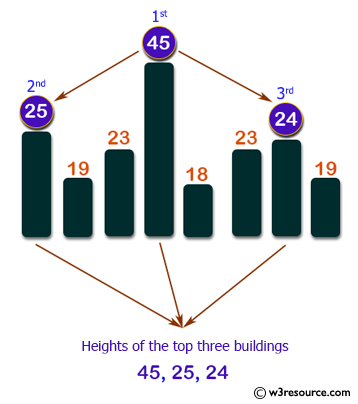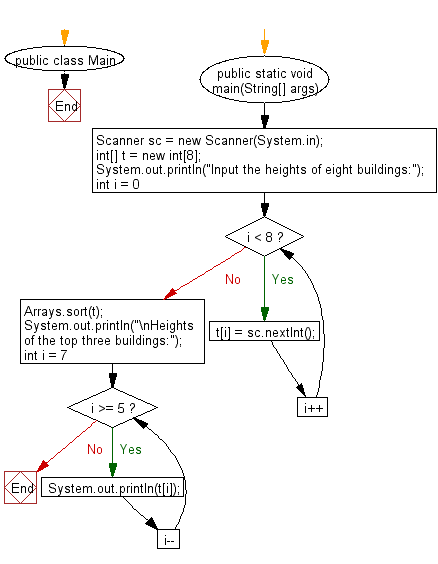# Java Exercises: Find heights of the top three building in descending order from eight given buildings

## Java Basic: Exercise-211 with Solution

Write a Java program to find heights of the top three building in descending order from eight given buildings.

Input:

0 ≤ height of building (integer) ≤ 10,000

Pictorial Presentation:Sample Solution:

Java Code:

``````import java.util.*;
public class Main {
public static void main(String[] args) {
Scanner sc = new Scanner(System.in);
int[] t = new int;
System.out.println("Input the heights of eight buildings:");
for(int i = 0; i < 8; i++){
t[i] = sc.nextInt();
}
Arrays.sort(t);
System.out.println("\nHeights of the top three buildings:");
for(int i = 7; i >= 5; i--){
System.out.println(t[i]);
}
}
}
```
```

Sample Output:

```Input the heights of eight buildings:
25 19 23 45 18 23 24 19

Heights of the top three buildings:
45
25
24
```

Flowchart:Java Code Editor:

What is the difficulty level of this exercise?

Test your Programming skills with w3resource's quiz.

﻿

## Java: Tips of the Day

Java: Anagrams

Generates all anagrams of a string.

```public static List<String> anagrams(String input) {
if (input.length() <= 2) {
return input.length() == 2
? Arrays.asList(input, input.substring(1) + input.substring(0, 1))
: Collections.singletonList(input);
}
return IntStream.range(0, input.length())
.mapToObj(i -> new SimpleEntry<>(i, input.substring(i, i + 1)))
.flatMap(entry ->
anagrams(input.substring(0, entry.getKey()) + input.substring(entry.getKey() + 1))
.stream()
.map(s -> entry.getValue() + s))
.collect(Collectors.toList());
}
```